# Lever physics

Be rest assured this is not a clash of titans; it's a fine combination of mathematics and physics. In this game, your goal is to balance the lever by putting all of the given objects onto it. At the start of each level, you will be given a lever which has labels to indicate the distances between the marked positions and the pivot of the lever. The objects and their weights are displayed at the bottom of the screen. You need to click and drag the objects onto the lever until the moments on both sides of the lever are the same, for example, if a 200g item is hanging under the 3m label on the left side, then the moment on the left is 200 x 3; and if on the right side a 300g item is hanging under the 1m label and a 100g item is hanging under the 3m label, then the moment on the right side is 300 x 1 + 100 x 3. In this case, the moments on the two sides are the same and therefore the lever will be balanced. If you have placed an item to an incorrect position, you can click and drag it back

## Related software (5)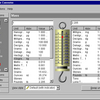## ABF Value Converter

ABF Value Converter is an application for measurement units conversion. This software easily converts various measurement values into any other possible ones. Inches to centimetres ...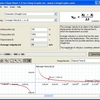## Physics Cheat Sheet

Physics Cheat Sheet is an interactive physics package that helps students solve and visualize numerous physics equations.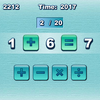## Quick Math

How quickly can you complete the equations with arithmetic symbols?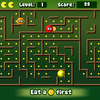## Math Man

Play Ghost Man with a mathematical twist!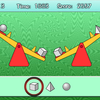## Seesaw Logic

Choose the heaviest object on the seesaw.